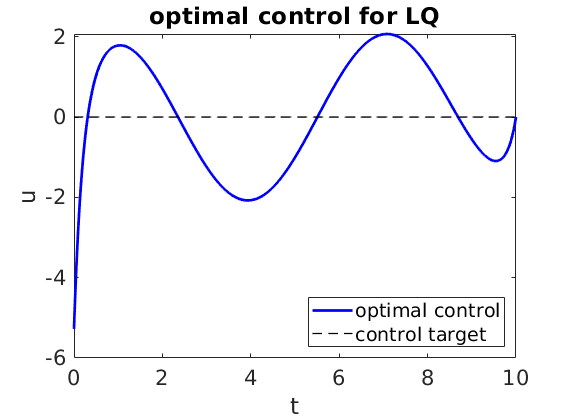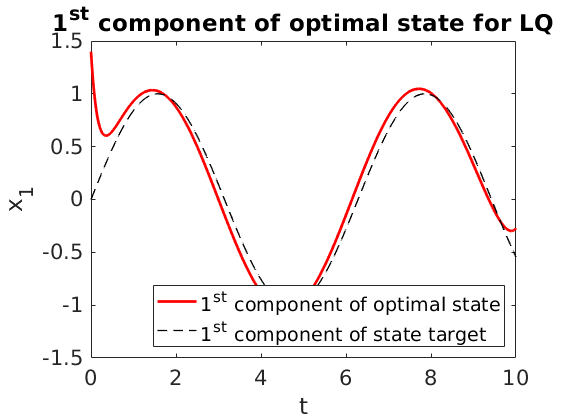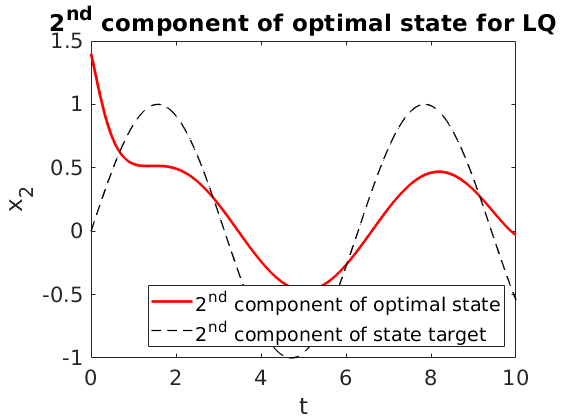# Riccati theory in LQ optimization with time-varying target

Author: - 21 April 2019

In this short tutorial, we explain how to use Riccati’s theory to solve an LQ control problem with targets.

We consider the optimal control problem:

where:

In the above control problem, $A \in M_{n \times n}$, $B \in M_{n \times m}$, $C \in M_{r\times n}$ and $D\in M_{r\times n}$. The control $u:[0,T]\longrightarrow R^m$, while the state $x:[0,T]\longrightarrow R^n$. The control target is $q\in C^1([0,T];R^m)$ and the state target is $z\in C^1([0,T];R^n)$. $\beta\geq 0$ and $\gamma\geq 0$ are positive parameters.

By the Direct Methods in the Calculus of Variations and strict convexity, the above problem admits an unique optimal control $u^T$. The corresponding optimal state is denoted by $x^T$.

We compute the optimal pair (optimal control, optimal state) by using the well-known Riccati’s theory (see, for instance, [1, Lemma 2.6] and [2, section 4.3]).

For further details regarding the algorithm, we refer to href=”https://github.com/DeustoTech/RiccatiLQ/blob/master/RiccatiAlgorithm.pdf” RiccatiAlgorithm /a

Take

A=[2,-1;-1,2];
B=[1;0];
C=eye(2);
D=zeros(2,2);
beta=26;
gamma=0;
x0=[1.4;1.4];
q=@(t)0;
z=@(t)[sin(t);sin(t)];
T=10;
Nt=1000;


We solve an LQ problem with the above data.

[ uopt, x] = lqtarget( A,B,C,D,beta, gamma, q, z, x0,T,Nt );Since the parameter $\beta$ is large enough and the control acts only on the first component of first equation

• the first component of the state is close to the target;

• the second component of the state is less close to the target;

• the control is far from its target.

The algorithm described in this guide can be employed to test the fulfillment of the turnpike property (see, for instance,  and ). In agreement with the theory, the turnpike effect is evident if:

• the targets are constants;

• $(A,B)$ is controllable;

• $(A,C)$ is observable, $\beta>0$ and $\gamma=0$;

• the time horizon $T$ is large enough.

 Alessio Porretta and Enrique Zuazua, Long time versus steady state optimal control, SIAM Journal on Control and Optimization, 51 (2013).

 Emmanuel Trelat, control optimal: theory & applications , Vuibert, 2008.

 Emmanuel Trelat and Enrique Zuazua, The turnpike property in finite-dimensional nonlinear optimal control, Journal of Differential Equations, 258 (2015).